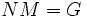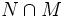# Abelian minimal normal subgroup and core-free maximal subgroup are permutable complements

## Statement

Suppose$G$ is a primitive group with core-free maximal subgroup$M$. Then, if$G$ has an Abelian minimal normal subgroup$N$, the following are true:

1.$NM = G$
2.$N$ and$M$ intersect trivially
3.$M \cong G/N$
4. The induced action of$N$ on$G/M$ by left multiplication, is equivalent to the regular action of a group on itself.

## Definitions used

### Core-free subgroup

Further information: Core-free subgroup

A subgroup of a group is termed core-free if its normal core in the whole group is trivial, or equivalently, if it does not contain any nontrivial normal subgroup of the whole group.

### Maximal subgroup

Further information: Maximal subgroup

A maximal subgroup of a group is a proper subgroup that is not contained in any bigger proper subgroup.

### Primitive group

Further information: Primitive group A primitive group is a group that possesses a core-free maximal subgroup.

## Facts used

1. Abelian normal subgroup and core-free subgroup generate whole group implies they intersect trivially

## Related facts

### Plinth theorem

Further information: plinth theorem

The plinth theorem is a similar result to the case of two distinct minimal nomral subgroups.

### More results under similar hypotheses

Proceeding further along the lines of this proof, one can show that:

## Proof

Given: A primitive group$G$ with core-free maximal subgroup$M$, and an Abelian minimal normal subgroup$N$

To prove:$N$ and$M$ are permutable complements:$NM = G$ and$N \cap M$ is trivial

Proof: Since$N$ is normal,$NM$ is a subgroup of$G$ containing$M$. Since$M$ is maximal, either$NM = M$ or$NM = G$. If$NM = M$, then$N \le M$, but this cannot happen because$M$ does not contain any nontrivial normal subgroup of$G$. Hence$NM = G$.

Thus$N$ is an Abelian normal subgroup and$M$ is a core-free subgroup that together generate$G$. Applying the fact (1) stated above,$N \cap M$ is trivial, so$N$ and$M$ are permutable complements.